Author: Oscar Cronquist Article last updated on January 18, 2023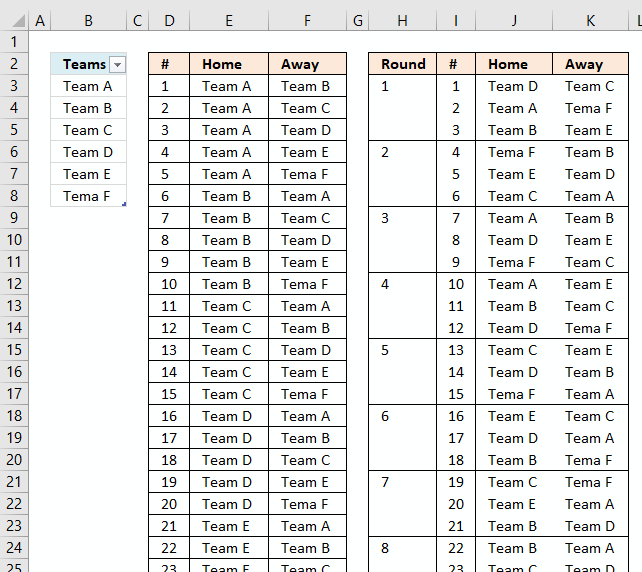Mark G asks in Create a random playlist in excel:

Can this example be modified to create a true round-robin draw?

i.e. using a 6 team scenario, for any round there will be three games/matches, but obviously, Team A could not compete against say Team B and Team C during that round as can occur using the above formula.

This example is based on a double round-robin in which every team plays all others in its league once at home and once away randomly.

A round-robin tournament or all-play-all tournament is a type of tournament in which each contestant meets all other contestants in turn. Source: Wikipedia

The picture above shows two playlists, the random playlist is the right one. Each round is divided using conditional formatting.

The worksheet is dynamic meaning you can have more or less teams and the worksheet will automatically generate a random playlist for you.

### How to use this workbook

The first playlist table is not random, it simply lists all possible matches for the entered team names. The second list is random.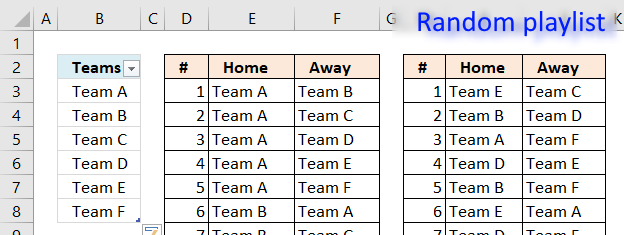You type the team names in column B in the Excel defined Table. It automatically expands when new values are entered, you don't need to adjust any formulas or cell references.

Simply type the next Team name in the first empty cell below the table.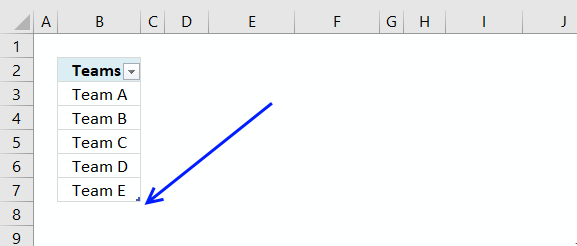#### Delete team names

To delete a name in the Excel defined table you need to select the cells you want to delete.

Then press with right mouse button on on the selected range.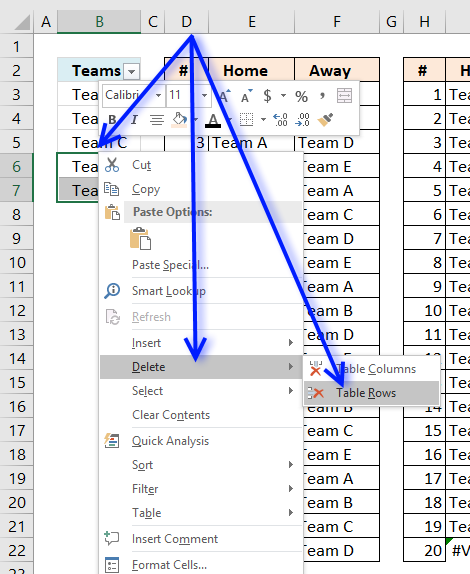Press with mouse on "Delete" and then press with left mouse button on "Table Rows".

If you accidentally press with left mouse button on "Table Columns", don't freak out. Simply press CTRL + z to undo.

#### Edit team names

You can edit the existing team names as well, the formulas are dynamic and return the correct names in the playlist instantly.

#### Generate the tournament playlist

There is a button to the right of the playlists.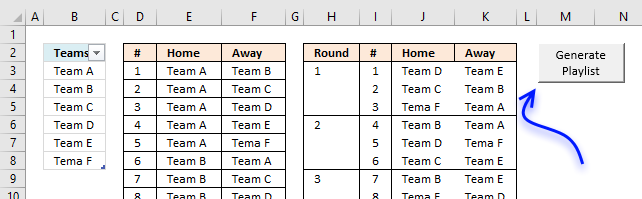Press with left mouse button on the button to automatically generate a new random playlist determined by the number of teams you have entered in column B.

When you have a playlist you are happy with copy it and paste it to a new worksheet.

### What does this workbook contain to make this possible?

Formula in cell D3 and below:

=IFERROR(SMALL(ROW(\$A\$1:INDEX(\$A\$1:\$A\$1000, COMBIN(ROWS(Table1[Teams]), 2)*2)), ROW(A1)), "")

Formula in cell E3 and below:

=IFERROR(INDEX(Table1[Teams],MATCH(FALSE, COUNTIF(\$E\$2:E2,Table1[Teams])=ROWS(Table1[Teams])-1,0)),"")

Formula in cell F3 and below:

=IF(E3<>"", INDEX(Table1[Teams], MATCH(0, COUNTIF(E3, Table1[Teams])+IFERROR(MATCH(Table1[Teams], IF(E3=\$E\$2:E2, \$F\$2:F2, ""), 0), 0), 0)), "")

Formula in cell H3 and below:

=IF((\$I3="")+(MOD(ROWS(\$A\$1:A1),INT(ROWS(Table1[Teams])/2))<>1),"",INT(ROWS(\$A\$1:A1)/(INT(ROWS(Table1[Teams])/2)))+1)

Formula in cell I3 and below:

=IFERROR(SMALL(ROW(\$A\$1:INDEX(\$A\$1:\$A\$1000, COMBIN(ROWS(Table1[Teams]), 2)*2)), ROW(A1)), "")

Formula in cell J3 and below:

=IF(I3<>"",INDEX(\$E\$3:\$F\$200,\$L3,COLUMN(A1)),"")

Formula in cell K3 and below:

=IF(I3<>"",INDEX(\$E\$3:\$F\$200,\$L3,COLUMN(A1)+1),"")

Hidden formula in cell L3 and below:

=IFERROR(IF(ROWS(Table1[Teams])>4, LARGE(ROW(\$A\$1:INDEX(\$A\$1:\$A\$1000,COMBIN(ROWS(Table1[Teams]),2)*2))*NOT(COUNTIF(\$L\$2:L2,  ROW(\$A\$1:INDEX(\$A\$1:\$A\$1000, COMBIN(ROWS(Table1[Teams]),2)*2))))*NOT(IFERROR(COUNTIF(OFFSET(J2:K2,0,0, -M3),ColE)+COUNTIF(OFFSET(J2:K2,0,0,-M3), ColF),0)), RANDBETWEEN(1,SUMPRODUCT(NOT(COUNTIF(\$L\$2:L2, ROW(\$A\$1:INDEX(\$A\$1:\$A\$1000, COMBIN(ROWS(Table1[Teams]),2)*2))))*NOT(IFERROR(COUNTIF(OFFSET(J2:K2,0,0,-M3), ColE)+COUNTIF(OFFSET(J2:K2, 0,0,-M3),ColF),0))))), LARGE(ROW(\$A\$1:INDEX(\$A\$1:\$A\$1000,COMBIN(ROWS(Table1[Teams]), 2)*2))*NOT(COUNTIF(\$L\$2:L2, ROW(\$A\$1:INDEX(\$A\$1:\$A\$1000,COMBIN(ROWS(Table1[Teams]), 2)*2)))),RANDBETWEEN(1,COMBIN(ROWS(Table1[Teams]), 2)*2+1-ROW(A1)))),"")

Hidden formula in cell M2:

=SUMPRODUCT(ISERROR(J3:K271)*1)

Hidden formula in cell M3 and cells below:

=MOD(ROW(OFFSET(\$A\$1, INT(ROWS(INDIRECT("Table1[Teams]"))/2)-2, 0))+ROW(A1), INT(ROWS(INDIRECT("Table1[Teams]"))/2))

VBA Macro
The random playlist sometimes returns error values, the following code verifies that no errors occur.

```Sub GeneratePlayList()
Application.ScreenUpdating = False
Do
Calculate
DoEvents
Loop While Worksheets("Sheet1").Range("M2") <> 0
Application.ScreenUpdating = True
End Sub
```

### Get the Excel filetrue-round-robin-draw7.xlsm